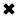# Capacitance Explained

Capacitance between two electrodes may be called for measurement purposes as "the charge stored per unit potential difference between them".

A useful device for storing electrical energy consists of two conductors in close proximity and insulated from each other, like a battery, where certain kinds of insulation material affects the battery's ability to store applied voltages. A simple example of such a storage device is the parallel plate capacitor. If positive charges with total charge +Q are deposited on one of the conductors and an equal amount of negative charge voltage -Q is deposited on the second conductor, the capacitor is said to stores charge Q. Such a device consists of two flat conducting plates, each of area A, parallel to each other and separated by a distance d.It depends on their surface area, spacing, applied votlages, and the character of the dielectric material or materials, which is affected by the electric field between them. The value of a capacitor, measured in farads or a convenient submultiple of this unit, will be influenced quite generally by temperature, pressure, or any ambient condition that changes the dimensions or spacing of the electrodes or the characteristics of the dielectric. The dielectric constant of a material is defined as the ratio of the unit of capacitance of a pair of electrodes, with the material occupying all the space affected by the field between them, to the capacitance of a capacitor of the same electrode configuration in vacuum.

PRINCIPLE OF CAPACITANCE

To understand how a charged capacitor stores energy and how current flows; consider the following charging process. With both plates of the capacitor initially uncharged, a small amount of negative charge is removed from the lower plate and placed on the upper plate. Thus, little work is required to make the lower plate slightly positive and the upper plate slightly negative. As the process is repeated, however, it becomes increasingly difficult to transport the same amount of negative charge, since the charge is being moved toward a plate that is already negatively charged and away from a plate that is positively charged. The negative charge on the upper plate repels the negative charge moving toward it, and the positive charge on the lower plate exerts an attractive force on the negative charge being moved away. Therefore, work has to be done to charge the capacitor.

Where and how is this energy stored? The inversely proportional negative charges on the upper plate are attracted toward the positive charges on the lower plate and could do work if they could leave the plate. Because they cannot leave the plate, however, the energy is stored. A mechanical analogy is the potential energy of a stretched spring. Another way to understand the energy stored in a capacitor is to compare an uncharged capacitor with a charged capacitor. In the uncharged capacitor, there is no electric field between the plates. In the charged capacitor, because of the positive and negative charges on the inside surfaces of the plates, there is an electric field between the plates with the field lines pointing from the positively charged plate to the negatively charged one. The energy stored is the energy that was required to establish the field. It is apparent that there is a nearly uniform electric field between the plates; the field becomes more uniform as the distance between the plates decreases and the area of the plates increases. In summary, the electric field is the change in the potential across a small distance in a direction perpendicular to an equipotential surface divided by that small distance. The upper plate is assumed to be at a potential of V(a) volts, and the lower plate at a potential of V(b) volts. The size of the electric field is:in volts per metre, where d is the separation of the plates. If the charged capacitor has a total charge of +Q on the inside surface of the lower plate (it is on the inside surface because it is attracted to the negative charges on the upper plate), the positive charge will be uniformly distributed on the surface with the value:in coulombs per metre squared. Equation (8) gives the electric field when the surface charge density is known as E = ak. This, in turn, relates the potential difference to the charge on the capacitor and the geometry of the plates. The result is:The unit used for capacity is the farad (F), named in honour of the English scientist Michael Faraday; one farad equals one coulomb per volt. In terms of ordinary electric and electronic equipment, the farad is enormous, and capacitors are generally rated in microfarads (ori.e microfarad equals 10-6 farad) or picofarads (10-12 farad). In equation, only the potential difference is involved. The potential of either plate can be set arbitrarily without altering the electric field between the plates. Often one of the plates is grounded - i.e., its potential is set at the Earth potential, which is referred to as zero volts.

##### Basic Electricity Handbook, Vol. 1• FULLY ILLUSTRATED
• GREAT FOR SCHOOL PROJECTS
• GREAT PRICE: \$5.99
• ...

This 100+ page e-book is a great guide for those who have a basic interest in the field of electricity. This well-illustrated e-book, coupled with some basic knowledge of electricity, will give you a broad theoretical background in this fundamental subject.

CONTENTS
• FUNDAMENTALS OF ELECTRICITY
• HOW DOES ELECTRICITY WORK?
• HOW IS ELECTRICITY GENERATED?
• WHERE DOES ELECTRICITY COME FROM?
• BASIC HOME WIRING
• ELECTRICITY FOR STUDENTS
• ENERGY SAVING TIPS

##### TRAINING EF COURSES

Content Community Connection
Top